# Evaluation

## Comparison of CFD Calculations with Experiments

A dramatic improvement in solution fidelity for DES compared to URANS, first reported by Shur et al. [‌22], was observed in the extensive cross-validation exercise carried out in the EU FLOMANIA project [‌4]. Figure 4 depicts the relative deviation from experimental drag achieved by DES and URANS within this work.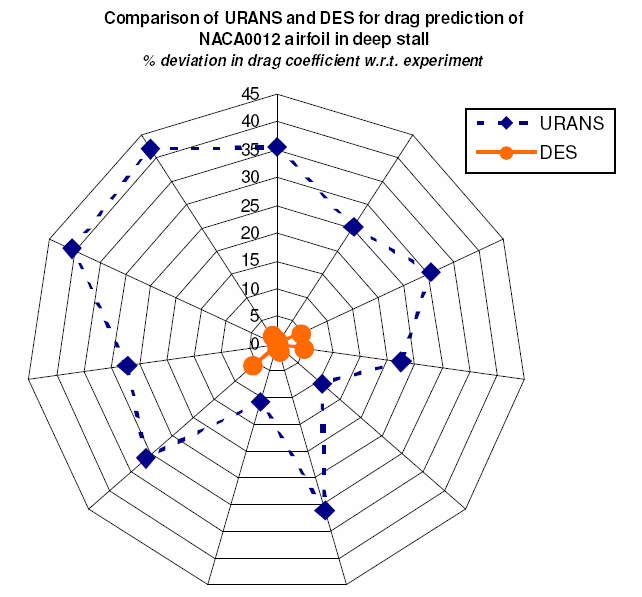Figure 4: Comparison of URANS and DES for the prediction of mean drag coefficient for the NACA0012 airfoil at α = 60°. Results of 11 different simulations conducted by different partners with different codes and turbulence models within the EU FLOMANIA project [‌4]. Experimental data cited by Hoerner [‌6] are used as reference.

The effect of spatial and temporal numerical schemes on DES was investigated for the NACA0012 case at α = 45° by Shur et  al. (2004) [‌23]. Using a localised "hybrid" convection scheme [‌29] (in which 4th order central differences are applied within the vortical wake region) and a 2nd order temporal integration was seen to resolve fine turbulent structures to a scale near to that of the local grid spacing. Switching the convection scheme to 3rd order upwind or, to a lesser extent, the temporal scheme to 1st order was seen to strongly damp the fine vortices in the wake (Figure 5). Correspondingly, a strong under- prediction of the Power Spectral Density (PSD) of the drag and lift forces at higher frequencies was observed. The effect on the mean forces and pressure distributions was however comparatively mild for this case.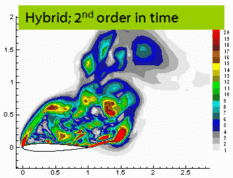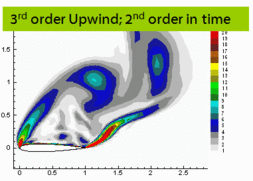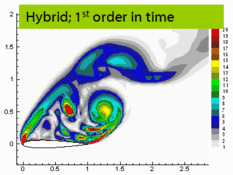Figure 5: Effect of different spatial and temporal numerical schemes on the resolved wake structures of the NACA0012 at α = 45° [‌23]. "Hybrid" refers to the localized blending between 4th order central and 3rd or 5th order upwind convection schemes proposed by Travin et al. [‌29]

Having clearly demonstrated the benefits of DES compared to URANS [‌4, 22] (Figure 4), no further URANS computations were carried out in the successor EU project DESider [‌5], and the focus shifted to cross-comparison of different turbulence-resolving approaches. Figure 6 compares flow visualizations from 3 simulations carried out with the use of different approaches (k – ω SST SAS and DES based on SA and CEASM RANS models) in the form of instantaneous fields of the vorticity magnitude. They reveal quite similar flow and turbulent structures thus supporting a marginal sensitivity of the simulations to the turbulence modelling approach and numerics used.

The same is to a major extent correct regarding the PSD of the lift coefficient and mean pressure distributions over the airfoil shown in Figure 7. Figure 7(a) also suggests that all the simulations are capable of predicting the experimental spectra, particularly the main shedding frequency and its harmonic, fairly well, whereas Figure 7(b) reveals a systematic difference between the predicted and measured pressure on the suction side. Note also that SAS results somewhat deviate from those of SA DES and are closer to the experiment. The same trend is observed for the integral lift and drag forces (Table 5). A concrete reason for the difference between the SAS and DES predictions is not clear but, in any case, it is not significant when compared to e.g. the differences between DES and URANS or between different URANS approaches (see Figure 4). This justifies the above conclusion on the weak sensitivity of the predictions to the turbulence modelling approach and numerics used in different turbulence-resolving simulations.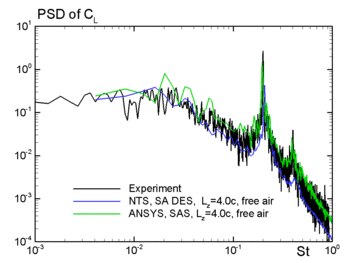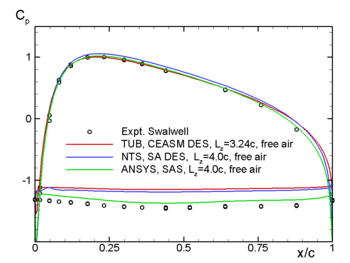(a) (b) Figure 7: Comparison of PSD of the lift coefficient (left) and mean pressure distributions over the airfoil (right) from three simulations with experimental data [‌27, 28]

 Partner and approach μ [Cl] Statistical 95% CI* μ [Cd] Statistical 95% CI* ANSYS (k – ω SST SAS, Lz=4) 0.915 ±0.017 1.484 ±0.030 EADS-M (SA DES, in wind-tunnel) 0.889 ±0.016 1.425 ±0.029 NTS (SA DES, Lz=4) 0.879 ±0.007 1.381 ±0.012 TUB (SA DES, Lz=4) 0.875 ±0.007 1.33 ±0.012 Experiment [‌24, 25] 0.931 ±0.004 1.517 ±0.008
*Confidence Interval

The parameters to which the results of the simulations turned out to be very sensitive include the span-size of the computational domain and time sample the turbulence statistics is calculated for. These parameters are therefore the most important in the sense of formulation the BPG for the considered UFR, and for this reason below we focus exactly on those studies which analyse their effect. These studies along with some original results related to this issue were summarized in [‌2], so the further presentation of the material closely follows this work.

### Effect of time sample

Obtaining accurate estimates of statistical quantities naturally requires a sufficient statistical sample. This raises fundamental questions concerning the engineering application of computationally expensive turbulence-resolving methods: how many time steps must be computed to estimate the statistical quantities of interest to the desired accuracy? The inverse perspective is just as important: what is the error bar on the statistical quantities when only a given number of time steps are affordable? The implications of this issue for the cost planning of industrial computational studies and the avoidance of false conclusions are clear.

An arbitrary section of the time series for the lift and drag coefficients from the experiment is shown in Figure 8. The quasi-periodic von Kármán vortex shedding cycles are seen (with a time period of roughly 5 convective time unites, (c / |U |) together with a strong low- frequency intermittency. This represents an apparently multi-modal behaviour of the flow, in which alternating weak and strong vortex shedding modes occur. The duration of each such mode as well as its initiation are random. As a result, it can intuitively be recognised that very long time samples will be required for accurate mean values of this flow.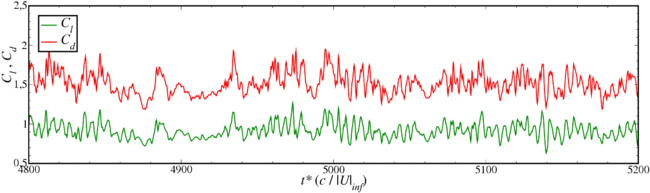Figure 8: Sample lift and drag coefficients time traces from the experimental data of Swalwell [‌27, 28], showing occurrence of weak and strong shedding behaviour.

A common method to obtain an impression of the time sample effect on the mean values is the computation of running averages. Indeed, this approach was applied in the DESider project study of this test case [‌5]. Figure 9 demonstrates such a running average for the experimental Cd trace, from which an indication is given that the mean Cd is still not reliable to the second decimal place after 4000 non-dimensional time units. Although useful in this respect, the running average technique does not provide any quantitative estimate of the statistical error. A potential peril therefore arises: were the data acquisition to have been ceased at $\left.t^{*}\right.$= 800, the strong and sudden drift that occurs shortly thereafter would not be anticipated. In order to get a quantitative estimate of the statistical error, a novel signal processing algorithm has been developed. The details of this technique have been summarised in [‌2] and published in more detail in [‌15]. For a given input signal, the magnitude of the statistical error as a function of sample length is estimated. The 95% confidence intervals for the experimental drag coefficient have been obtained in this way and plotted over the running average in Figure 9.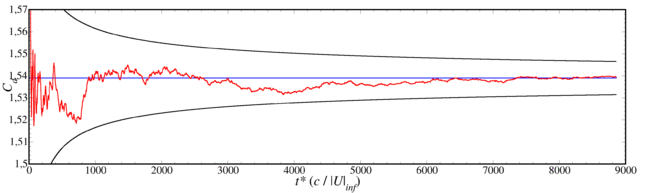Figure 9: Running average of experimental drag coefficient [‌27, 28], giving an impression of the level of drift that occurs even after long averaging times (red curve). Envelope of 95% confidence interval arising from statistical error (black curves).

### Span-size effects

The spanwise separation between the periodic boundaries to the computational domain has been varied between Lz = 1c and 4c in the calculations of NTS and TUB, allowing the influence of this user parameter to be studied. Figure 10 and Figure 11 draw upon all DES results employing a periodic spanwise domain and all applicable experimental data to provide an overview. The 95% confidence intervals due to the statistical error estimated using the technique mentioned in the preceding section [‌2, 15][‌2] are portrayed in the figures by error brackets.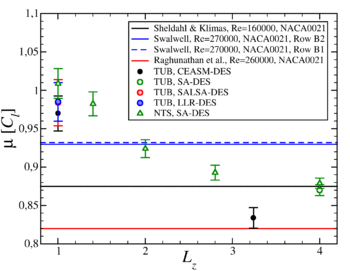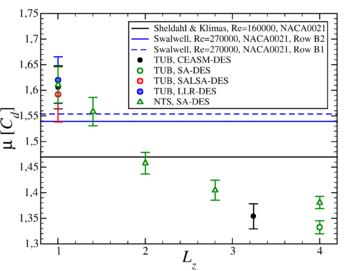Figure 10: Trends of mean lift and drag coefficients against Lz for the NACA0021 at α = 60° and Re = 270000 (unless otherwise stated), including bars representing the statistical 95% confidence interval.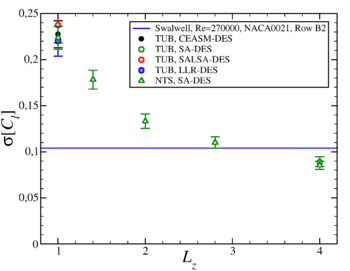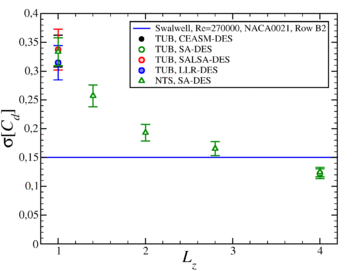Figure 11: Trends of standard deviation of the lift and drag coefficients against Lz for the NACA0021 at α = 60° and Re = 270000, bars representing the statistical 95% confidence interval.

The effect of a narrow span size is to increase all quantities depicted. The effect is indeed pronounced, far outweighing the statistical error and the model/code dependency. When sufficient statistical samples are computed, Lz hence constitutes the most influential source of error. It is to be expected that this finding would apply to nominally 2D bluff bodies in general.

The plots of Figure 10 and Figure 11 suggest that the Lz influence becomes negligible at values of around 4c for this test case, although further computations at still larger Lz values would strictly be required to demonstrate this. An indication that the required Lz is strongly case-specific is given by comparison with the results of Guenot  for the lower α = 45° case. In that case, the Lz effect was seen to settle at lower values of Lz ≈ 2c. It is believed that the wider wake is responsible for the larger Lz required for our α = 60° case.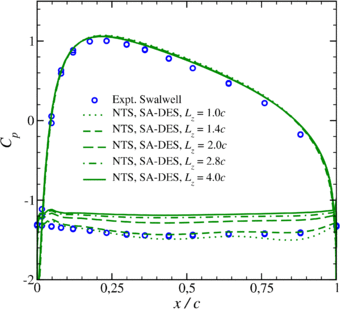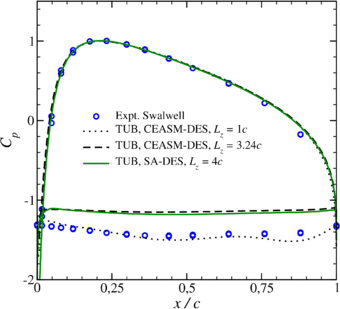Figure 12: The effect of Lz on the mean Cp distribution. Comparison of NTS SA-DES results (left) and TUB CEASM and SA-DES results (right) with the experimental data of Swalwell [‌27,28]

The mean surface pressure coefficient, Cp, plotted in Figure 12, corroborates the trends shown by Fig. 10 and shows that the Lz effect is manifested in a strong variation of the base pressure on the downstream (upper) surface of the airfoil. The high comparability between the left-hand and right-hand plots demonstrates the negligible influence of different solvers and underlying RANS models. The paradox that the agreement with experiment degrades with increasing Lz was initially a source of concern. However, the observation of a strong scatter between the experiments (particularly visible in Fig. 10) reduces this. Furthermore, the conclusion to be reached from Fig. 10 is that increasing Lz brings the mean Cl inside the experimental range and in excellent agreement with the Sheldahl & Klimas data. For the mean Cd however, the agreement is less positive. This could in part be because the Cd value from the Raghunathan et al., experiment is unknown: Assuming that the lift-to-drag ratio of 0.60 observed in the other experiments applies, this could be estimated to lie in the region of Cd ≈ 1.37.

Unlike the mean quantities, the effect of increasing Lz on the prediction of unsteady quantities is definitely positive: as seen in Figure 11, a dramatic improvement of agreement with the data on the standard deviation of the integral forces is achieved at longer Lz values. More detail is offered by the PSD of Cl, plotted in Figure 13. Particularly regarding the lowest frequencies, which are associated with the long-wavelength intermittency, the agreement of the broadband energy is strongly improved at Lz = 4c. The sharp spectral peak at St = 0.2 represents the vortex shedding frequency which well agrees with the experiment. However a tangible disagreement with the data in the energy of the peak which is more clearly seen in plots of the energy pre-multiplied by frequency (not shown) is somewhat troublesome.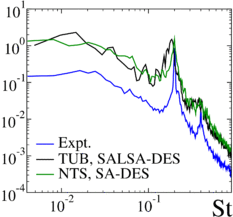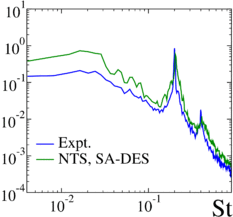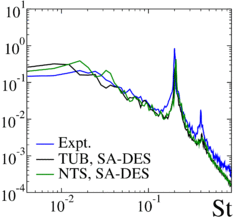Figure 13: PSD of Cl compared between NTS and TUB DES results with varying Lz and the Swalwell experimental data [‌27,28].

A natural statistical quantity to examine in such an investigation is the two-point correlation of velocity in the spanwise direction, ${R_{ii}(z)}$, where i represents the u, v and w velocity components. These are plotted in Figure 14 at four probe positions in the near wake for TUB's computations at Lz = 1c and Lz = 4c. For the u and v components, the correlations settle to lower values in the wider domain, however they clearly remain above zero. Such long spanwise correlation lengths naturally arise due to the strong spanwise coherence of the large-scale vortex shedding. For the w component a different behaviour is seen: in the narrow domain the correlation consistently reaches a negative value, implying an anti-phase behaviour. For the longer domain however, the w correlation settles around zero. It is therefore suggested that ${R_{ww}(z)}$could provide a generic test for sufficient span size in practice. This would however require more testing to confirm.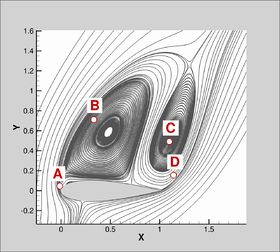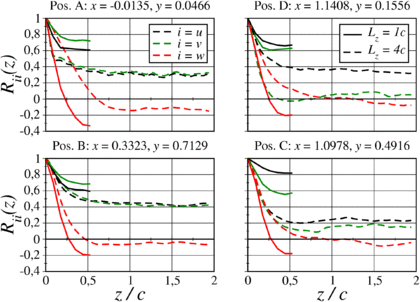Figure 14: Two-point spanwise correlations of u, v and w compared for Lz = 1c (TUB CEASM-DES) and Lz = 4c (TUB SA-DES) at the probe locations shown in the left-hand figure.

Finally, further insight into the span size effect can be obtained from instantaneous visualisations of the resolved vortex structures (Figure 15). The streamwise rib vortices and spanwise von Kármán vortices maintain a high degree of orthogonality in the narrow spanwise domain. In contrast, these exhibit less orthogonality with spanwise dislocations and higher degrees of slant apparent in the wider domain.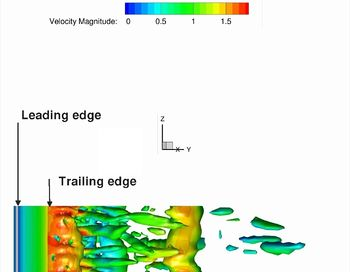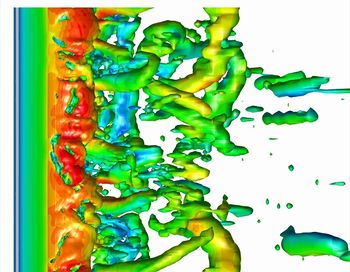Figure 15: Instantaneous snapshots of resolved vortical structures compared between Lz = 1c (TUB CEASM-DES) and Lz = 4c (TUB SA-DES) calculations. Isosurfaces of λ2 = -4 [‌7] shaded with velocity magnitude, view from below.

### Effect of wind tunnel walls

It was suspected that the surrounding wind tunnel walls, neglected in the spanwise-periodic computations, may contribute to the discrepancy with the Swalwell experiments. This was the motivation for the EADS simulation including the wind tunnel walls. The mean surface Cp and PSD of Cl plotted in Figure 16 indicate a negligible impact of the tunnel walls. The time-averaged force coefficients give a similar message: The Cl = 0.89 ± 0.016 and Cd = 1.43 ± 0.029 from the computation with wind tunnel walls do not differ significantly from the NTS periodic computation (Cl = 0.88 ± 0.007 and Cd = 1.38 ± 0.012). Although the comparison is unfortunately not direct (employing different Lz, solvers, grid resolution, and time step), the experience of various related investigations implies that these factors have a negligible influence for this test case.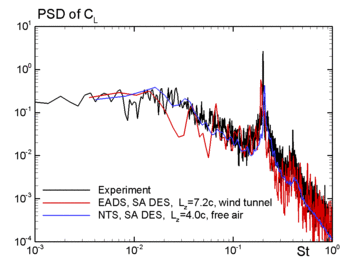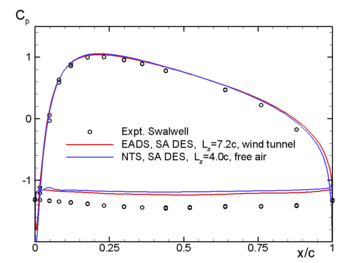Figure 16: Comparison of PSD of Cl (left) and mean surface Cp (right) between the spanwise-periodic NTS computation (Lz = 4c) and the EADS computation with side walls (Lz = 7.2c) and with the experimental data of Swalwell [‌27,28].

1. This drift arises as the flow switches to a protracted bout of strong shedding behaviour at around t* = 800.
2. A software implementing this algorithm for the estimation of statistical error and the detection of initial transient is commercially available: Contact info@cfd-berlin.com for details.
3. Lz is not expected to have a large effect for values above around 4c

Contributed by: Charles Mockett; Michael Strelets — CFD Software GmbH and Technische Universitaet Berlin; New Technologies and Services LLC (NTS) and St.-Petersburg State Polytechnic University